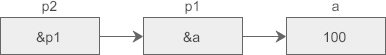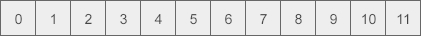XUHAO’S 博客 Always hungry to keep learning.

# C 语言指针概念的理解（四）

2016-08-22

## 指针##

``````#include <stdio.h>

int main ()
{
int  var1;
char var2;

printf("var1 变量的地址： %x\n", &var1  );
printf("var2 变量的地址： %x\n", &var2  );

return 0;
}
``````

``````var1 变量的地址： bff5a400
var2 变量的地址： bff5a3f6
``````

## 什么是指针？

``````type *name;
``````

``````int    *ip;    /* 一个整型的指针 */
double *dp;    /* 一个 double 型的指针 */
float  *fp;    /* 一个浮点型的指针 */
char   *ch     /* 一个字符型的指针 */
``````

## 如何使用指针？

• 使用指针时会频繁进行以下几个操作：定义一个指针变量、把变量地址赋值给指针、访问指针变量中可用地址的值。这些是通过使用一元运算符 * 来返回位于操作数所指定地址的变量的值。下面的实例涉及到了这些操作：
``````#include <stdio.h>
//指针
//指针存储的是变量的内存地址
//内存地址，系统给数据分配的编号（门牌号）

void main(){
int i = 90;
//指针变量，创建一个int类型的指针
int* p = &i; //p的值就是i这个变量的内存地址
printf("%#x\n",p);

float f = 89.5f;
//创建一个float类型的指针
float *fp = &f;
printf("%#x\n", fp);

system("pause");
}
``````

``````0xeffb30
0xeffb18

``````
• 对指针存的地址指向的变量进行操作
``````#include <stdio.h>

void change(int* p){
*p = 300;
}

//变量名，对内存空间上的一段数据的抽象
void main(){
int i = 90;
//i = 89;
//创建一个int类型的指针
int *p = &i;
//输出地址
printf("p的地址：%#x\n",&p);
printf("i的地址：%#x\n",&i);
printf("i的值为：%d\n", i);
//间接赋值 i = 200;

//对p存的地址指向的变量进行操作
//*p = 200;
//change(p);
change(&i);  // int *p = &i;
printf("i的值为：%d\n",i);

system("pause");
}
``````

``````p的地址：0x6ffdb0
i的地址：0x6ffdbc
i的值为：90
i的值为：300

``````

## C中的NULL 指针

``````#include <stdio.h>

int main ()
{
int  *ptr = NULL;

printf("ptr 的值是 %x\n", ptr  );

return 0;
}
``````

``````ptr 的值是 0
``````

``````#include <stdio.h>

int main(int argc, char const *argv[]) {

char *s = "hello";

if (!s) {
fprintf(stderr, "s is null\n");
} else {
fprintf(stderr, "%s\n", s);
}

if (s == NULL) {
fprintf(stderr, "s is null\n");
} else {
fprintf(stderr, "%s\n", s);
}

return 0;
}
``````

## C中的二级指针（多级指针）``````int a =100;
int *p1 = &a;
int **p2 = &p1;
``````

``````int ***p3 = &p2;
``````

``````int ****p4 = &p3;
``````

``````#include <stdio.h>
int main(){
int a =100;
int *p1 = &a;
int **p2 = &p1;
int ***p3 = &p2;
printf("%d, %d, %d, %d\n", a, *p1, **p2, ***p3);
printf("&p2 = %#X, p3 = %#X\n", &p2, p3);
printf("&p1 = %#X, p2 = %#X, *p3 = %#X\n", &p1, p2, *p3);
printf(" &a = %#X, p1 = %#X, *p2 = %#X, **p3 = %#X\n", &a, p1, *p2, **p3);
return 0;
}
``````## C中指针的运算

``````
#include <stdio.h>
//指针的运算，一般在数组遍历时才有意义，基于数组在内存中线性排列的方式
void main(){
//数组在内存中连续存储
int ids[] = { 78, 90, 23, 65, 19 };
//数组变量名：ids就是数组的首地址
printf("%#x\n",ids);
printf("%#x\n",&ids);
printf("%#x\n",&ids);
//指针变量
int *p = ids;
printf("%d\n",*p);
//指针的加法
p++; //p++向前移动sizeof(数据类型)个字节
printf("p的值:%#x\n", p);
//p--;
printf("%d\n", *p);
getchar();
}
``````

``````0x5ff7d0
0x5ff7d0
0x5ff7d0
78
p的值:0x5ff7d4
90

``````

``````#include <stdio.h>
int main(){
int a = 1, b = 2, c = 3;
int *p = &c;
int i;
for(i=0; i<8; i++){
printf("%d, ", *(p+i) );
}
return 0;
}
``````

``````3, -858993460, -858993460, 2, -858993460, -858993460, 1, -858993460,
``````

## C中的指针数组

``````dataType *arrayName[length];
``````

]的优先级高于*，该定义形式应该理解为：

``````dataType *(arrayName[length]);
``````

``````#include <stdio.h>
int main(){
int a = 16, b = 932, c = 100;
//定义一个指针数组
int *arr = {&a, &b, &c};//也可以不指定长度，直接写作 int *parr[]
//定义一个指向指针数组的指针
int **parr = arr;
printf("%d, %d, %d\n", *arr, *arr, *arr);
printf("%d, %d, %d\n", **(parr+0), **(parr+1), **(parr+2));
return 0;
}
``````

``````16, 932, 100
16, 932, 100
``````

arr 是一个指针数组，它包含了 3 个元素，每个元素都是一个指针，在定义 arr 的同时，我们使用变量 a、b、c 的地址对它进行了初始化，这和普通数组是多么地类似。

parr 是指向数组 arr 的指针，确切地说是指向 arr 第一个元素的指针，它的定义形式应该理解为int * ( * parr)，括号中的 * 表示 parr 是一个指针，括号外面的int * 表示 parr 指向的数据的类型。arr 第一个元素的类型为 int *， 所以在定义 parr 时要加两个 * （星号）（MD语法自动识别其他的格式了）。

``````#include <stdio.h>
void main(){
int uids;
//高级写法
//int i = 0;
//for (; i < 5; i++){
//	uids[i] = i;
//}
//早些版本的写法
int* p = uids;
printf("%#x\n",p);
int i = 0; //i是数组元素的值
for (; p < uids + 5; p++){
*p = i;
i++;
}

getchar();
}
``````

## C中的指针与二维数组

``````int a = { {0, 1, 2, 3}, {4, 5, 6, 7}, {8, 9, 10, 11} };
``````

``````0   1   2   3
4   5   6   7
8   9  10  11
``````C语言中的二维数组是按行排列的，也就是先存放 a 行，再存放 a 行，最后存放 a 行；每行中的 4 个元素也是依次存放。数组 a 为 int 类型，每个元素占用 4 个字节，整个数组共占用 4×(3×4) = 48 个字节。

C语言允许把一个二维数组分解成多个一维数组来处理。对于数组 a，它可以分解成三个一维数组，即 a、a、a。每一个一维数组又包含了 4 个元素，例如 a 包含 a、a、a、a。

``````int (*p) = a;
``````

int *p; //定义一个指针数组，该数组中每个元素是一个指针，每个指针指向哪里就需要程序中后续再定义了。

int (*p); //定义一个数组指针，该指针指向含4个元素的一维数组（数组中每个元素是int型）。

``````区分int *p[n]; 和int (*p)[n]; 就要看运算符的优先级了。
int *p[n]; 中，运算符[ ]优先级高，先与p结合成为一个数组，再由int*说明这是一个整型指针数组。
int (*p)[n]; 中( )优先级高，首先说明p是一个指针，指向一个整型的一维数组。
``````

• p指向数组 a 的开头，也即第 0 行；p+1前进一行，指向第 1 行。
• *(p+1)表示取地址上的数据，也就是整个第 1 行数据。注意是一行数据，是多个数据，不是第 1 行中的第 0 个元素，下面的运行结果有力地证明了这一点：
``````#include <stdio.h>
int main(){
int a = { {0, 1, 2, 3}, {4, 5, 6, 7}, {8, 9, 10, 11} };
int (*p) = a;
printf("%d\n", sizeof(*(p+1)));
return 0;
}
``````

``````16
``````

``````  *(p+1)单独使用时表示的是第 1 行数据，放在表达式中会被转换为第 1 行数据的首地址，也就是第 1 行第 0 个元素的地址，因为使用整行数据没有实际的含义，编译器遇到这种情况都会转换为指向该行第 0 个元素的指针；就像一维数组的名字，在定义时或者和 sizeof、& 一起使用时才表示整个数组，出现在表达式中就会被转换为指向数组第 0 个元素的指针。

``````
``````* ( *(p+1)+1) 表示第 1 行第 1 个元素的值。很明显，增加一个 * 表示取地址上的数据。
``````

``````a+i == p+i
a[i] == p[i] == *(a+i) == *(p+i)
a[i][j] == p[i][j] == *(a[i]+j) == *(p[i]+j) == *(*(a+i)+j) == *(*(p+i)+j)
``````

``````#include <stdio.h>
int main(){
int a={0,1,2,3,4,5,6,7,8,9,10,11};
int(*p);
int i,j;
p=a;
for(i=0; i<3; i++){
for(j=0; j<4; j++) printf("%2d  ",*(*(p+i)+j));
printf("\n");
}
return 0;
}
``````

`````` 0   1   2   3
4   5   6   7
8   9  10  11
``````

``````int *(p1);  //指针数组，可以去掉括号直接写作 int *p1;
int (*p2);  //二维数组指针，不能去掉括号
``````

## C中的函数指针

``````returnType (*pointerName)(param list);
``````

returnType 为函数返回值类型，pointerNmae 为指针名称，param list 为函数参数列表。

( )的优先级高于*，第一个括号不能省略，如果写作returnType *pointerName(paramlist); 就成了函数原型，它表明函数的返回值类型为returnType *。

``````#include <stdio.h>
#include <Windows.h>

int msg(char* msg,char* title){
MessageBox(0,msg,title,0);
return 0;
}
void main(){
//msg();
printf("%#x\n",msg);
printf("%#x\n",&msg);
//函数指针
//函数返回值类型，函数指针的名称，函数的参数列表
int(*fun_p)(char* msg, char* title) = msg;
fun_p("消息内容","标题");

getchar();
}
``````## 总结：

``````- int *p;	    p 可以指向 int 类型的数据，也可以指向类似 int arr[n] 的数组。
- int **p;	    p 为二级指针，指向 int * 类型的数据。
- int *p[n];	p 为指针数组。[ ] 的优先级高于 *，所以应该理解为 int *(p[n]);
- int (*p)[n];	p 为二维数组指针。
- int *p();	    p 是一个函数，它的返回值类型为 int *。
- int (*p)();	p 是一个函数指针，指向原型为 int func() 的函数。
``````